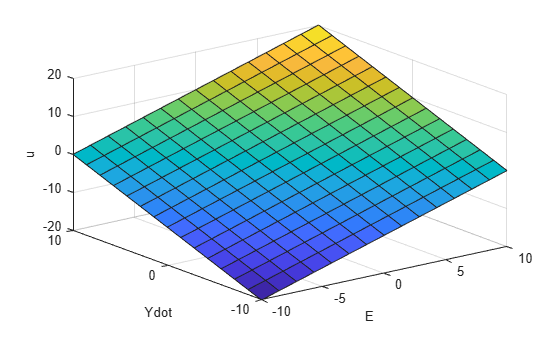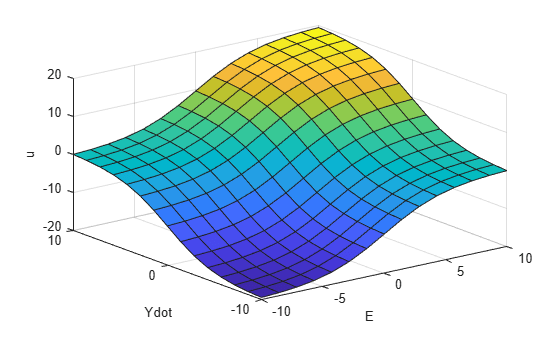Main Content

# Implement Fuzzy PID Controller in Simulink Using Lookup Table

This example shows how to implement a fuzzy inference system for nonlinear PID control using a 2-D Lookup Table block.

### Overview

A fuzzy inference system (FIS) maps given inputs to outputs using fuzzy logic. For example, a typical mapping of a two-input, one-output fuzzy controller can be depicted in a 3-D plot. The plot is often referred to as a control surface plot.For control applications, typical FIS inputs are the error (`e(k)`) and change of error (`e(k)-e(k-1)`), `E` and `CE` respectively in the control surface plot. The FIS output is the control action inferred from the fuzzy rules, `u` in the surface plot. Fuzzy Logic Toolbox™ provides commands and apps for designing a FIS for a desired control surface. You can then simulate the designed FIS using the Fuzzy Logic Controller block in Simulink®.

You can often approximate nonlinear control surfaces using lookup tables to simplify the generated code and improve execution speed. For example, you can replace a Fuzzy Logic Controller block in Simulink with a set of Lookup Table blocks, one table for each output defined in the FIS. You can compute the data used in the lookup table using the `evalfis` command.

For this example, you design a nonlinear fuzzy PID controller for a plant in Simulink. The plant is a single-input, single-output system in discrete time. The design goal is to achieve good reference tracking performance.

```Ts = 0.1; Plant = c2d(zpk([],[-1 -3 -5],1),Ts); ```

You also implement the fuzzy inference system using a 2-D lookup table that approximates the control surface and achieves the same control performance.

### Fuzzy PID Controller Structure

The fuzzy controller in this example is in the feedback loop and computes PID-like actions using fuzzy inference. Open the Simulink model.

```open_system('sllookuptable') ```The fuzzy PID controller uses a parallel structure as shown in the Fuzzy PID subsystem. For more information, see . The controller is a combination of fuzzy PI control and fuzzy PD control.

```open_system('sllookuptable/Fuzzy PID') ```The fuzzy PID controller uses the change of the output `-(y(k)-y(k-1))`, instead of change of error `e(k)-e(k-1)`, as the second input signal to the FIS. Doing so prevents the step change in reference signal from directly triggering the derivative action. The two gain blocks, `GCE` and `GCU`, in the feed forward path from `r` to `u`, ensure that the error signal `e` is used in proportional action when the fuzzy PID controller is linear.

### Design Conventional PID Controller

The conventional PID controller in this example is a discrete-time PID controller with Backward Euler numerical integration in both the integral and derivative actions. The controller gains are `Kp`, `Ki`, and `Kd`.

```open_system('sllookuptable/Conventional PID') ```Similar to the fuzzy PID controller, the input signal to the derivative action is `-y(k)`, instead of `e(k)`.

You can tune the PID controller gains manually or using tuning formulas. In this example, obtain the initial PID design using the `pidtune` command from Control System Toolbox™.

Define the PID structure, tune the controller, and extract the PID gains.

```C0 = pid(1,1,1,'Ts',Ts,'IF','B','DF','B'); C = pidtune(Plant,C0) [Kp,Ki,Kd] = piddata(C); ```
```C = Ts*z z-1 Kp + Ki * ------ + Kd * ------ z-1 Ts*z with Kp = 30.6, Ki = 25.2, Kd = 9.02, Ts = 0.1 Sample time: 0.1 seconds Discrete-time PID controller in parallel form. ```

### Design Equivalent Linear Fuzzy PID Controller

By configuring the FIS and selecting the four scaling factors, you can obtain a linear fuzzy PID controller that reproduces the control performance of the conventional PID controller.

First, configure the fuzzy inference system so that it produces a linear control surface from inputs `E` and `CE` to output `u`. The FIS settings are based on design choices described in :

• Use a Sugeno style fuzzy inference system with default inference methods.

• Normalize the ranges of both inputs to [-10 10].

• Use triangular input membership functions that overlap their neighbor functions at a membership value of 0.5.

• Use an output range of [-20 20].

• Use constant output membership functions.

Construct the fuzzy inference system.

```FIS = sugfis; ```

Define input variable `E`.

```FIS = addInput(FIS,[-10 10],'Name','E'); FIS = addMF(FIS,'E','trimf',[-20 -10 0],'Name','Negative'); FIS = addMF(FIS,'E','trimf',[-10 0 10],'Name','Zero'); FIS = addMF(FIS,'E','trimf',[0 10 20],'Name','Positive'); ```

Define input `CE`.

```FIS = addInput(FIS,[-10 10],'Name','CE'); FIS = addMF(FIS,'CE','trimf',[-20 -10 0],'Name','Negative'); FIS = addMF(FIS,'CE','trimf',[-10 0 10],'Name','Zero'); FIS = addMF(FIS,'CE','trimf',[0 10 20],'Name','Positive'); ```

Define output variable `u` with `constant` membership functions.

```FIS = addOutput(FIS,[-20 20],'Name','u'); FIS = addMF(FIS,'u','constant',-20,'Name','LargeNegative'); FIS = addMF(FIS,'u','constant',-10,'Name','SmallNegative'); FIS = addMF(FIS,'u','constant',0,'Name','Zero'); FIS = addMF(FIS,'u','constant',10,'Name','SmallPositive'); FIS = addMF(FIS,'u','constant',20,'Name','LargePositive'); ```

Define the following fuzzy rules:

1. If `E` is negative and `CE` is negative, then `u` is `-20`.

2. If `E` is negative and `CE` is zero, then `u` is `-10`.

3. If `E` is negative and `CE` is positive then `u` is `0`.

4. If `E` is zero and `CE` is negative, then `u` is `-10`.

5. If `E` is zero and `CE` is zero, then `u` is `0`.

6. If `E` is zero and `CE` is positive, then `u` is `10`.

7. If `E` is positive and `CE` is negative, then `u` is `0`.

8. If `E` is positive and `CE` is zero, then `u` is `10`.

9. If `E` is positive and `CE` is positive, then `u` is `20`.

```ruleList = [1 1 1 1 1; % Rule 1 1 2 2 1 1; % Rule 2 1 3 3 1 1; % Rule 3 2 1 2 1 1; % Rule 4 2 2 3 1 1; % Rule 5 2 3 4 1 1; % Rule 6 3 1 3 1 1; % Rule 7 3 2 4 1 1; % Rule 8 3 3 5 1 1]; % Rule 9 FIS = addRule(FIS,ruleList); ```

While you implement your FIS from the command line in this example, you can alternatively build your FIS using the Fuzzy Logic Designer app.

Plot the linear control surface.

```gensurf(FIS) ```Determine scaling factors `GE`, `GCE`, `GCU`, and `GU` from the `Kp`, `Ki`, and `Kd` gains of by the conventional PID controller. Comparing the expressions of the traditional PID and the linear fuzzy PID, the variables are related as follows:

• `Kp` = `GCU` * `GCE` + `GU` * `GE`

• `Ki` = `GCU` * `GE`

• `Kd` = `GU` * `GCE`

Assume that the maximum reference step is `1`, and thus the maximum error `e` is `1`. Since the input range of `E` is [-10 10], set `GE` to `10`. You can then solve for `GCE`, `GCU`, and `GU`.

```GE = 10; GCE = GE*(Kp-sqrt(Kp^2-4*Ki*Kd))/2/Ki; GCU = Ki/GE; GU = Kd/GCE; ```

### Implement Fuzzy Inference System Using 2-D Lookup Table

The fuzzy controller block has two inputs (`E` and `CE`) and one output (`u`). Therefore, you can replace the fuzzy system using a 2-D lookup table.

To generate a 2-D lookup table from your FIS, loop through the input universe, and compute the corresponding output values using `evalfis`. Since the control surface is linear, you can use a few sample points for each input variable.

```Step = 10; E = -10:Step:10; CE = -10:Step:10; N = length(E); LookUpTableData = zeros(N); for i=1:N for j=1:N % Compute output u for each combination of sample points. LookUpTableData(i,j) = evalfis(FIS,[E(i) CE(j)]); end end ```

View the fuzzy PID controller using 2-D lookup table.

```open_system('sllookuptable/Fuzzy PID using Lookup Table') ```The only difference compared to the Fuzzy PID controller is that the Fuzzy Logic Controller block is replaced with a 2-D Lookup Table block.

When the control surface is linear, a fuzzy PID controller using the 2-D lookup table produces the same result as one using the Fuzzy Logic Controller block.

### Simulate Closed-Loop Response in Simulink

The Simulink model simulates three different controller subsystems, namely Conventional PID, Fuzzy PID, and Fuzzy PID using Lookup Table, to control the same plant.

Run the simulation. To compare the closed-loop responses to a step reference change, open the scope. As expected, all three controllers produce the same result.

```sim('sllookuptable') open_system('sllookuptable/Scope') ```### Design Fuzzy PID Controller with Nonlinear Control Surface

Once you have a linear fuzzy PID controller, you can obtain a nonlinear control surface by adjusting your FIS settings, such as its style, membership functions, and rule base.

For this example, design a steep control surface using a Sugeno-type FIS. Each input set has two terms (`Positive` and `Negative`), and the number of rules is reduced to four.

Construct the FIS.

```FIS = sugfis; ```

Define input `E`.

```FIS = addInput(FIS,[-10 10],'Name','E'); FIS = addMF(FIS,'E','gaussmf',[7 -10],'Name','Negative'); FIS = addMF(FIS,'E','gaussmf',[7 10],'Name','Positive'); ```

Define input `CE`.

```FIS = addInput(FIS,[-10 10],'Name','CE'); FIS = addMF(FIS,'CE','gaussmf',[7 -10],'Name','Negative'); FIS = addMF(FIS,'CE','gaussmf',[7 10],'Name','Positive'); ```

Define output `u`.

```FIS = addOutput(FIS,[-20 20],'Name','u'); FIS = addMF(FIS,'u','constant',-20,'Name','Min'); FIS = addMF(FIS,'u','constant',0,'Name','Zero'); FIS = addMF(FIS,'u','constant',20,'Name','Max'); ```

Define the following rules:

1. If `E` is negative and `CE` is negative, then `u` is `-20`.

2. If `E` is negative and `CE` is positive, then `u` is `0`.

3. If `E` is positive and `CE` is negative, then `u` is `0`.

4. If `E` is positive and `CE` is positive, then `u` is `20`.

```ruleList = [1 1 1 1 1;... % Rule 1 1 2 2 1 1;... % Rule 2 2 1 2 1 1;... % Rule 3 2 2 3 1 1]; % Rule 4 FIS = addRule(FIS,ruleList); ```

View the 3-D nonlinear control surface. This surface has a higher gain near the center of the `E` and `CE` plane than the linear surface has, which helps reduce the error more quickly when the error is small. When the error is large, the controller becomes less aggressive to avoid possible saturation.

```gensurf(FIS) ```Before starting the simulation, update the lookup table with the new control surface data. Since the surface is nonlinear, to obtain a sufficient approximation, add more sample points.

```Step = 1; E = -10:Step:10; CE = -10:Step:10; N = length(E); LookUpTableData = zeros(N); for i=1:N for j=1:N % Compute output u for each combination of sample points. LookUpTableData(i,j) = evalfis(FIS,[E(i) CE(j)]); end end ```

Run the simulation.

```sim('sllookuptable') ```Compared with the traditional linear PID controller (the response curve with large overshoot), the nonlinear fuzzy PID controller reduces the overshoot by 50%. The two response curves from the nonlinear fuzzy controllers almost overlap, which indicates that the 2-D lookup table approximates the fuzzy system well.

```bdclose('sllookuptable') % Closing model also clears its workspace variables. ```

### Conclusion

You can approximate a nonlinear fuzzy PID controller using a lookup table. By replacing a Fuzzy Logic Controller block with Lookup Table blocks in Simulink, you can deploy a fuzzy controller with simplified generated code and improved execution speed.

### References

 Xu, J. X., Hang, C. C., Liu, C. "Parallel structure and tuning of a fuzzy PID controller." Automatica, Vol. 36, pp. 673-684. 2000.

 Jantzen, J. Tuning of Fuzzy PID Controllers, Technical Report, Dept. of Automation, Technical University of Denmark. 1999.

Download now